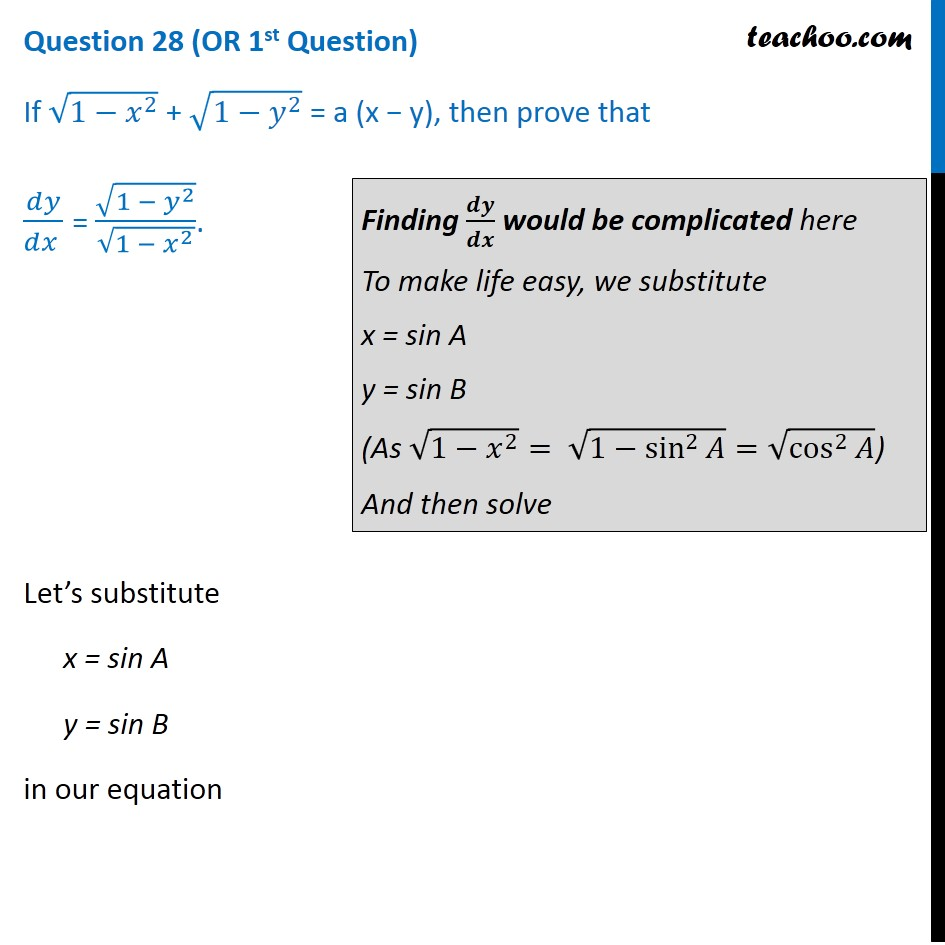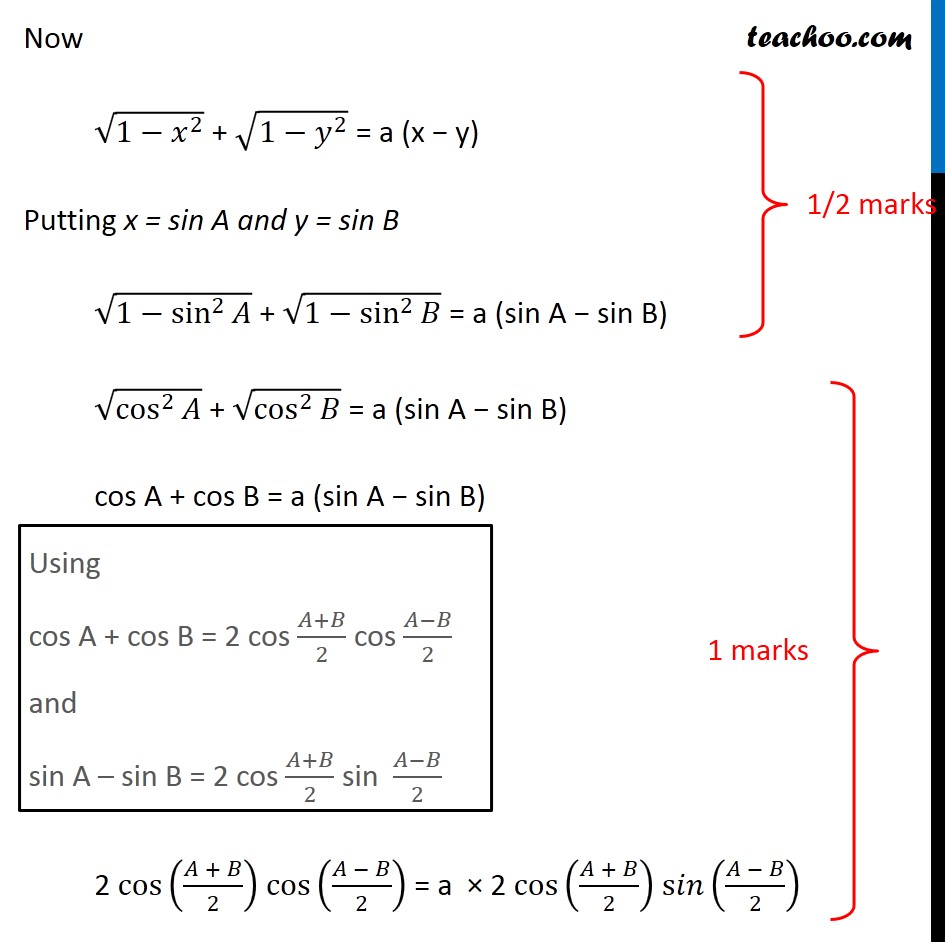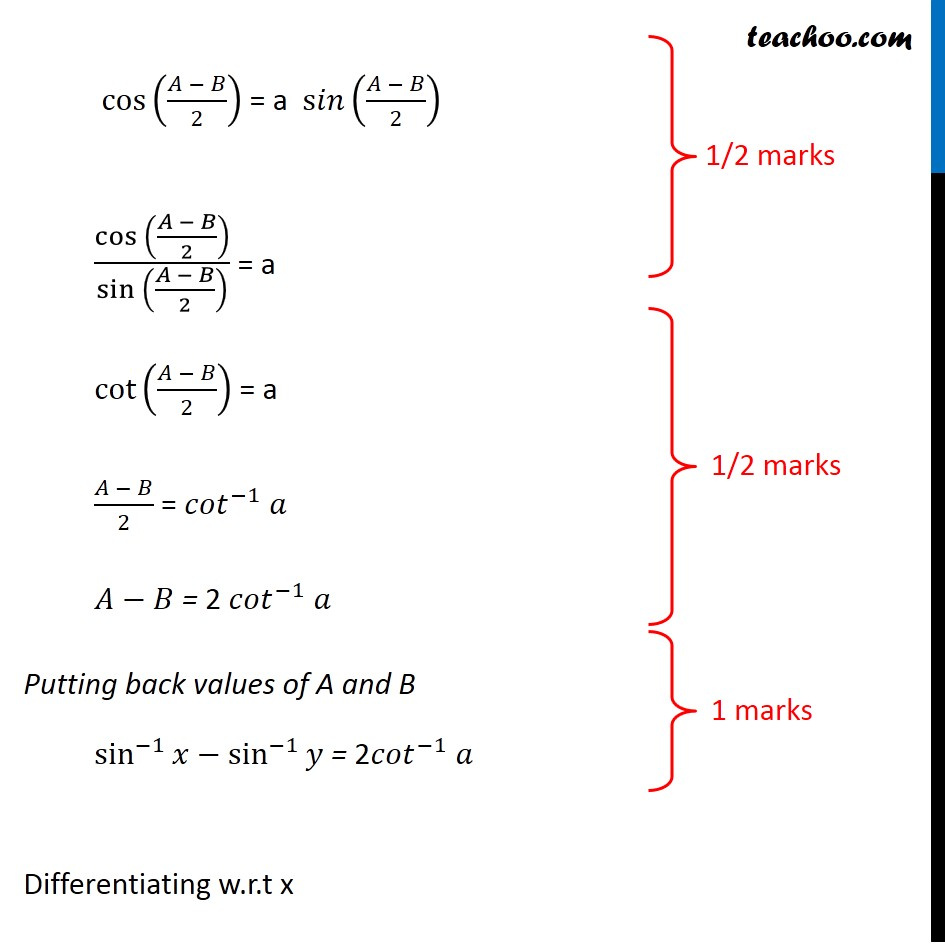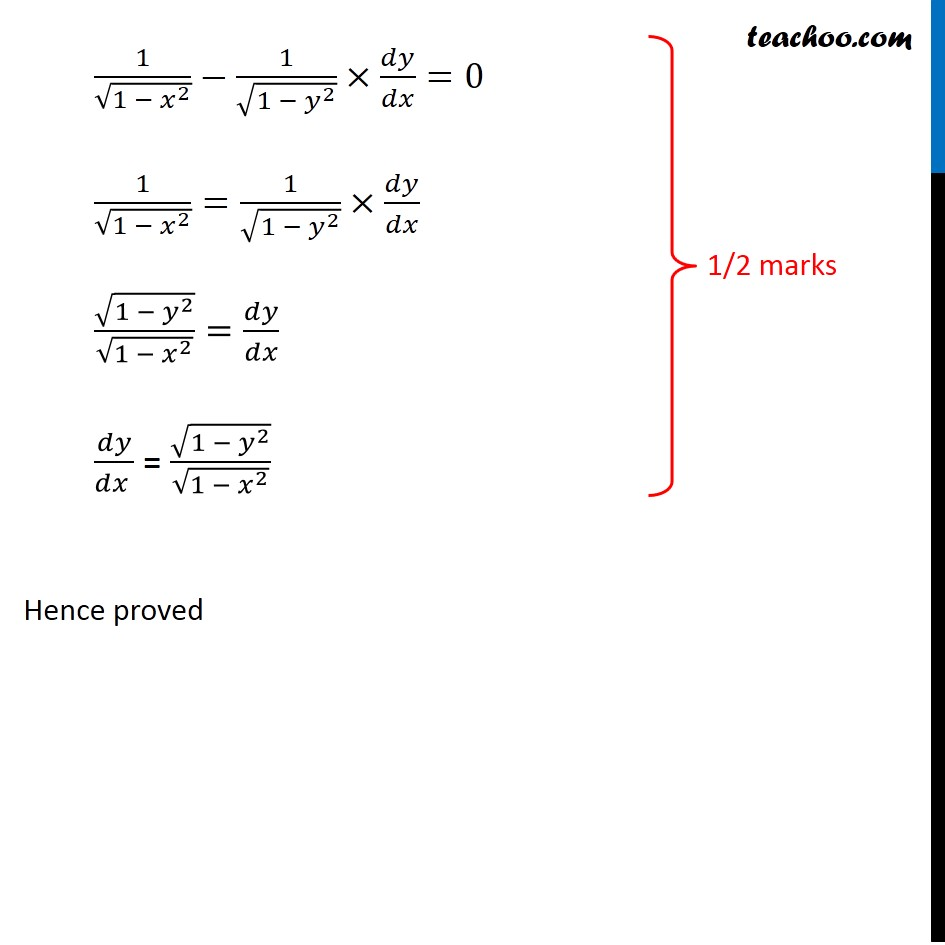CBSE Class 12 Sample Paper for 2020 Boards

Class 12
Solutions of Sample Papers and Past Year Papers - for Class 12 Boards

## dy/dx = √(1 - y 2 )/√(1 - x 2 ).Learn in your speed, with individual attention - Teachoo Maths 1-on-1 Class

### Transcript

Question 28 (OR 1st Question) If √(1−𝑥^2 ) + √(1−𝑦^2 ) = a (x − y), then prove that 𝑑𝑦/(𝑑𝑥 ) = √(1 − 𝑦^2 )/√(1 − 𝑥^2 ). Finding 𝒅𝒚/𝒅𝒙 would be complicated here To make life easy, we substitute x = sin A y = sin B (As √(1−𝑥^2 )= √(1−sin^2⁡𝐴 )=√(cos^2⁡𝐴 )) And then solve Let’s substitute x = sin A y = sin B in our equation Now √(1−𝑥^2 ) + √(1−𝑦^2 ) = a (x − y) Putting x = sin A and y = sin B √(1−sin^2⁡𝐴 ) + √(1−sin^2⁡𝐵 ) = a (sin A − sin B) √(cos^2⁡𝐴 ) + √(cos^2⁡𝐵 ) = a (sin A − sin B) cos A + cos B = a (sin A − sin B) Using cos A + cos B = 2 cos (𝐴+𝐵)/2 cos (𝐴−𝐵)/2 and sin A – sin B = 2 cos (𝐴+𝐵)/2 sin (𝐴−𝐵)/2 2 cos⁡((𝐴 + 𝐵)/2) cos⁡((𝐴 − 𝐵)/2) = a × 2 cos⁡((𝐴 + 𝐵)/2) s𝑖𝑛⁡((𝐴 − 𝐵)/2) cos⁡((𝐴 − 𝐵)/2) = a s𝑖𝑛⁡((𝐴 − 𝐵)/2) 〖cos 〗⁡((𝐴 − 𝐵)/2)/〖sin 〗⁡((𝐴 − 𝐵)/2) = a cot⁡((𝐴 − 𝐵)/2) = a (𝐴 − 𝐵)/2 = 〖𝑐𝑜𝑡〗^(−1) 𝑎 𝐴−𝐵 = 2 〖𝑐𝑜𝑡〗^(−1) 𝑎 Putting back values of A and B sin^(−1)⁡𝑥−sin^(−1)⁡𝑦 = 2〖𝑐𝑜𝑡〗^(−1) 𝑎 Differentiating w.r.t x 1/√(1 − 𝑥^2 )−1/√(1 − 𝑦^2 )×𝑑𝑦/𝑑𝑥=0 1/√(1 − 𝑥^2 )=1/√(1 − 𝑦^2 )×𝑑𝑦/𝑑𝑥 √(1 − 𝑦^2 )/√(1 − 𝑥^2 )=𝑑𝑦/𝑑𝑥 𝑑𝑦/(𝑑𝑥 ) = √(1 − 𝑦^2 )/√(1 − 𝑥^2 ) Hence proved